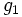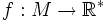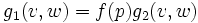# Conformally equivalent metrics

(diff) ← Older revision | Latest revision (diff) | Newer revision → (diff)

## Definition

### Symbol-free definition

Two Riemannian metrics on a differential manifold are termed conformally equivalent if one of them can be obtained as a scalar function times the other one. In other words, at each point, one metric is simply a constant times the other metric (the constant may vary from point to point).

### Definition with symbols

Let$g_1$ and$g_2$ be two Riemannian metrics on a differential manifold$M$. Then we say that$g_1$ is conformally equivalent to$g_2$ if there is a scalar function$f:M \to \mathbb{R}^*$ such that for any$p \in M$, and tangent vectors$v,w \in T_pM$:$g_1(v,w) = f(p)g_2(v,w)$